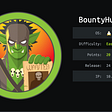# The Science (math) behind Aimbot

While i was doing my own research about making aimbot, i found the tutorials in the internet are either inconsistent, the techniques differ from each other, others are hard to understand, others are just straight up not working. So i learnt it myself and now, i will make this writeup for future hackers that also have troubles learning and understanding the concept behind aimbot. I will not be going on how to make the hack, i will only show the math behind the hack. For the demo, i will be using csgo but this can be applied on any game as well.

So how does an aimbot work. For me, aimbot are just a bunch of calculation of triangles. Now you must be asking, what triangle, where triangle, and how triangle? Well, lets take this image as an example

This is an example of a top down view i made. We want to aim to the target player

Now where is the triangle here? in here, we can actually make 2 right triangles.

Now with either of these two right triangle, we can then use the pythagorean theorem to calculate the angle required to aim to the player

When calculating the angle in the z axis , we will also be using the same concept.

Now we know the logic behind how aimbot works, i have to clarify other things before we start coding. In 3d games, the position is stored inside a Vector 3. A vector 3 is a group of 3 floats containing x,y, and z.

Other thing we need to know is how cameras are rotated. Cameras are commonly rotated by rotating on an axis. When we move a camera left or right, we rotate by the z axis, which is also called yaw. When we moved up or down, we rotate by the y or x axis which is also called pitch

Now that we have those out of the way, lets start coding.

First, we have to make a few structs first to make our life easier

We made a Vec3 struct that have 3 floats called x, y, and z. We also make a Rotate struct with the pitch and yaw.

First, we will store the player of our local player and the target player in the world and pass them to a function we will make later that i called CalcAngle which will then return the pitch and yaw rotation that we need to aim to the target. I will not show how to get the position of the player and the target. It is outside the scope of this writeup.

Now lets make our CalcAngle.

CalcAngle will accept two arguments, the position of our player, and the position of the target and will return the pitch and yaw required for the aimbot. We will first calculate the yaw. Now lets first get a, b and c. We will be using this photo as a reference

So how do we get a and b? simple, a is the distance from our local player to the target player in the x axis and b is the distance from our local player to the target player in the y axis. We can get the distance by subtracting the target position to our player position

Now to get c, we will use pythagoream theorem c² = a² + b².

Now that we have a,b, and c. We can now calculate the angle. If you listen to your highschool math lesson, you should know it already, but since i dont, lets look it up online instead.

Here, we can see that there are multiple ways to get the angle that we need but he circled the arctan so we will choose that. Arctan in c++ is atan that is included in the cmath library. So the value of the angle is the atan of opp/adj. opp is b and adj is a.

Its return value is in radians but we need it in degrees. To convert it to degrees, according to geeksforgeeks , we need to multiply it by 180/pi. And that will be our yaw.

Then, we will assign the return value of CalcAngle to our view angle

Now if we try it in game, you can see that we will suddenly snap our aim to the target player.

If you play around it, you can see that if our a is negative, our aimbot will aim in the opposite direction. I found out that it can be solved by adding 180 into the angle so we aim in the right direction. I dont know why it happens but the solution work so ¯\_(ツ)_/¯.

Here’s our new code

Now lets calculate the pitch, it should be nearly the same but we will be using the y axis as the a and the z axis as the b. Now in csgo, facing upward is -90 degrees and facing downward is 90 degrees so we have to make our angle negative too.

Now if we run this, our aimbot should also auto aim in the z axis.

Now we have a working aimbot. After making this writeup, i noticed that we never actually used c, you can remove it if you want.

Some more info: In the time of writing this writeup, i found out that there is a function called atan2. You can use it in yaw to remove the if and else if statement and it will magically work. Also, there’s a bug where in certain positions, the pitch will just aim at wrong angle. Idk yet why it happen. If you know, please do let me know

This is the end of this writeup. I do not promote cheating and this writeup is for educational purpose only. I hope you learn something. I posted the source code in github https://github.com/noobexploiter/LEARN-GAME-HACKING/tree/main/aimbot

--

--

--

## More from Brandon Roldan

Love podcasts or audiobooks? Learn on the go with our new app.

## Using Slack features efficiently## Let’s slow it down now…## Hosting websites inside AKS with Cloudflare and Let’s Encrypt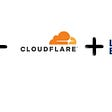## Optimized Solutions: Frequency Counters## How to squash last X commits after they have been pushed## IOST Chrome Extension iWallet v0.2.9 Update Official Release## Solster IDO Launchpad and Staking Testnet Launch Summary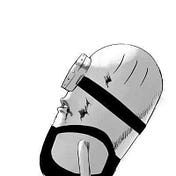## picoCTF write up: vault-door-1## HTB: Sense Writeup w/o Metasploit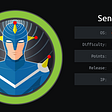## Log4Shell — You should know about it.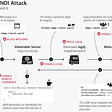## Hack The Box —BountyHunter Writeup